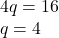## 3p + 4q = 22 10p + 12 q = 68 What is p and what is q (Similtaneous equations)

Question

3p + 4q = 22
10p + 12 q = 68
What is p and what is q
(Similtaneous equations)

in progress 0
2 months 2021-07-27T17:16:11+00:00 2 Answers 5 views 0

q=4

p=2

Step-by-step explanation:

3p+2q=14

10p+6q=44

10(3p+2q=14)

3(10p+6q=44)

30p+20q=140-

30p+18q=132

2q=8

2q/2=8/2

q=4

3p+2*4=14

3p+8=14

3p=14-8

3p/3=6/3

p=2

hope this helps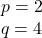Step-by-step explanation:

One is given the following system of equations,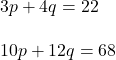The fastest method to solve a system of equations is the method of elimination. This process is manipulating one of the equations, by multiplying or diving it by a value, such that one of the coefficients variables in the equation is the additive inverse of the like term in the other equation. That way, when one adds the equations, one of the variables cancels out. Then one can solve for the other term. Finally, one can back sovle by substituting the value of the solved variable into one of the equations and simplifying to find the value of the other variable.Manipulate the first equation so that the variable (q) cancels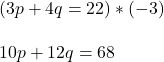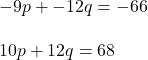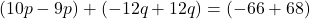Simplify,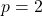Backsovle for the variable (p). Substitute the values of (p) into one of the original equations. Then simplify and use inverse operations to solve for the variable (q).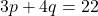Substitute,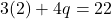Simplify,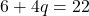Inverse operations,